Next Article in Journal
Inkjet Printing in Liquid Media: Intra-Volumetric Drop Coalescence in Polymers
Next Article in Special Issue
Study of Birefringence and Stress Distribution of SiO2 Film Optical Waveguide on Silicon Wafer
Open AccessEditor’s ChoiceArticle

# Development of Belt-Type Microstructure Array Flexible Mold and Asymmetric Hot Roller Embossing Process Technology

Department of Mechanical and Energy Engineering, National Chiayi University, No. 300, Syuefu Road, Chiayi City 600, Taiwan
Coatings 2019, 9(4), 274; https://doi.org/10.3390/coatings9040274
Received: 18 March 2019 / Revised: 19 April 2019 / Accepted: 20 April 2019 / Published: 22 April 2019
(This article belongs to the Special Issue Design, Manufacturing and Measurement of Optical Film Coatings)

## Abstract

This study proposed the belt-type microstructure array flexible mold designed hot roller embossing process technology. An extrusion molding system was integrated with belt-type hot roller embossing process technology and, deriving the asymmetric principle as the basis of prediction, designed a belt-type microstructure array hot roller embossing process system. This study first focused on the design and manufacturing of a belt-type hot roller embossing process system (roll to belt-type). It then carried out system integration and testing, along with the film extrusion system, to fabrication microstructure array production. Hot embossing was used to replicate the array of the plastic micro lens as the microstructure mold. The original master mold was fabricated with micro electromechanical technology and the PC micro lens array as the microstructure (inner layer) film using the gas-assisted hot embossing technology. A microstructure composite belt and magnetic belt were produced on the hot roller embossing by an innovated coated casting technique. The forming accuracy of the belt-type microstructure array flexible mold hot roller embossing process and the prediction precision of numerically simulated forming were discussed. The proposed process technology is expected to effectively reduce the process cycle time with the advantages of being a fast and continuous process.

## 1. Introduction

In recent years, due to the vigorous development of the semiconductor industry and the continuous progress of high technology, commercialized testing instruments, optical communication products, and consumer electronics products are facing the trend of miniaturization, integration, and multifunctional development. Traditional reflective and refractive micro structural components are very limited due to their special properties. It is difficult to develop general miniaturizing processes, due to high cost and difficult mass production. The plastic micro optical components of the same function are inexpensive and easily produced in quantity, and can be integrated with the existing optoelectronic components to form a micro optical mechanical and electrical system [1,2,3]. Due to the wide application of plastic polymer embossing and forming technology [4,5,6] in microstructure array components and vast market demands, two kinds of embossing and replicating form technology (plane type and roller type) are commonly used. However, compared to the plane embossing technology, the roller type embossing technology [7,8,9,10,11,12,13,14,15,16,17] has the advantages of continuous mass production and shortened embossing duration. In 1997, Gale  used electroplating nickel mold technology to duplicate the extremely thin nickel mold, in order to achieve mass replication of the structure. The technology adopted a roller coated with nickel mold and roll-to-roll production concept [19,20,21]. However, during the rolling process, due to a too-fast rolling speed (roller speed), the parts actually touched by the imprint are only the quick contacts of roller to roller. This might easily cause insufficient time for the holding pressure. In this case, the imprint is indefinite or the rebound causes insufficient width and depth, or in the general rolling process, incomplete contact problems between the roll to roll and roller central section often occur. At the same time, general roller embossing is formed on the roller or coated on the roller with a microstructure. However, as a tight adherence is difficult, mold displacement and warping occur frequently during rolling. In addition, roller imprint technology is applied to hot pressed nanoimprint lithography, and its mold belongs to the non-transparent rigid mold, which requires precise high temperature and high pressure processing equipment. Therefore, the process is slow and the cost of the mold is high. The high cost of precision roller embossing equipment is the main deficiency of this kind of process. If a soft mold is used for roller embossing, it has insufficient strength and is not resistant to high temperature. In order to improve the above shortcomings, this study addressed the above problems, and developed the belt-type microstructure array micro hot rolling process technology. The proposed technology is expected to continuously replicate the microstructure of the belt on the surface of polymer film by using the continuous extrusion process of the extruder, continuous film extrusion, and the continuous rolling process of the belt rolling system. The effect of the holding pressure of the embossing of the belt could be continued, which is expected to efficiently solve the rebound caused by too fast embossing and indefinite embossing width. In addition to effectively utilizing the magnetic attraction between magnetic rollers to perform high density imprinting, the high precision rolling action is assisted by adjusting the appropriate gas to form pressure.

## 2. Asymmetric Principle for Roller Embossing System

#### 2.1.1. General Expressions

At first, given the feature size and geometric position of the tip, we can completely describe the tip shape and configuration. That is, we can get the equations that represent the surface of the tip shape, which are attached on the model. Suppose there exists a point $C i$ on the surface of the model $M$, the point $C i$ can be represented by a position vector $R i , R$, as show in Equation (1).
Here the subscript $R$ is the reference frame, it is a fixed frame attached on the machine platform. In our system, the machine platform is fixed at all times, but the substrate can be lifted.
Then let us see what happens after we rotate the model. Given $φ$ and $θ$, we can get two rotation operators, one concerns the effect of $φ$ rotation, another concerns the effect of $θ$ rotation.
Rotation operator $O φ ; R → M ( 1 )$, which describes a point transforms into a new position, and consequently a new coordinate accompanies it after the model’s proceeding $φ$ rotation, as shown in Equation (2).
$O φ ; R → M ( 1 ) = ( cos ( φ ) sin ( φ ) 0 − sin ( φ ) cos ( φ ) 0 0 0 1 ) ,$
where $R$ is the reference frame and $M$ is the frame attached on the model. Rotation operator $O φ ; M ( 1 ) → M ( 2 )$, which describes a point transforms into a new position, and consequently a new coordinate accompanies it after the model proceeding $θ$ rotation, as show in Equation (3).
$O φ ; M ( 1 ) → M ( 2 ) = ( cos ( θ ) 0 0 0 1 0 − sin ( θ ) 0 1 ) ,$
Let us proceed to the operation of the two operators. Step 1 is the $φ$ rotation, that is $O φ ; R → M ( 1 )$, on the point $C i$. We can see after the model $M$ $φ$ rotation, the point $C i$ goes to a new position, it is represented by a position vector $R i , M ( 1 )$, as show in Equation (4).
$R i , M ( 1 ) = O φ ; R → M ( 1 ) · R i , R ,$
The second step is that we proceed with $θ$ rotation, that is $O θ ; M ( 1 ) → M ( 2 )$, continuously after the $φ$ rotation. Then the point $C i$, represented by the position vector $R i , M ( 1 )$, should go to another new position, described by a position vector $R i , M ( 2 )$, as show in Equation (5).
$R i , M ( 2 ) = O θ ; M ( 1 ) → M ( 2 ) · R i , M ( 1 ) = O θ ; M ( 1 ) → M ( 2 ) · O φ ; R → M ( 1 ) · R i , R ,$
Thus, we can get the new coordinate of some point $C i$, which lies on the surface of the tip (or model) at all times but is transformed into a new space position after the two rotation operations, $O φ ; R → M ( 1 )$ and $O θ ; M ( 1 ) → M ( 2 )$. Here we have to understand that the coordinate of any point, say $C i$, regardless of whether it is transformed or not, is always described in terms of machine platform reference frame, $R$.
To double check the correctness of the above expressions, we define a rotation operator $O − φ ; M ( 1 ) → R$, describing the model $− φ$ rotation, as show in Equation (6).
$O − φ ; M ( 1 ) → R = ( cos ( − φ ) sin ( − φ ) 0 − sin ( − φ ) cos ( − φ ) 0 0 0 1 ) = ( cos ( φ ) − sin ( φ ) 0 sin ( φ ) cos ( φ ) 0 0 0 1 )$
If there exists a point that is represented by a vector $R i , M ( 1 )$, then after the point undergoes the rotation operator $O − φ ; M ( 1 ) → R$, it should go back to the original position $R i , R$. That means operators $O φ ; R → M ( 1 )$ and $O − φ ; M ( 1 ) → R$ are inverse to each other, as show in Equation (7).
$O φ ; R → M ( 1 ) · O − φ ; M ( 1 ) → R = O − φ ; M ( 1 ) → R · O φ ; R → M ( 1 ) = 1 ,$

#### 2.1.2. Case One: Cylinder-Shape Tip

Here we consider the case of the tip, the mold is cylinder-shape. At first, let us see the surface equation of the cylinder-shape tip and the plane equation of substrate. We assume the surface equation of the cylinder-shape tip is:
Here we do not show the explicit form of this cylinder-shape tip surface equation. The plane equation of the substrate is:
$ψ s u b s t r a t e = { 0 · ( x − 0 ) + 0 · ( y − 0 ) + 1 · ( z − 0 ) = 0 z = 0 }$, substrate is not lifted, and $ψ s u b s t r a t e = { 0 · ( x − 0 ) + 0 · ( y − 0 ) + 1 · ( z − 0 ) = 0 z = Δ h }$, substrate has been lifted a distance $Δ h$.
Thus, after solving the equations for $ψ c y l i n d e r$ and $ψ s u b s t r a t e$, we can get the imprinted intersection contour, which is shown on the substrate. The imprinted contour equation, as show in Equation (8):
The contour is a cycle (as a = b) or an ellipse (as a ≠ b).

#### 2.1.3. Case Two: Sphere-Shape Tip

Similar to the case of the cylinder-shape tip, at first, let us see the surface equation of the sphere-shape tip and the plane equation of substrate. We assume the surface equation of the cylinder-shape tip is:
Here we do not show the explicit form of this sphere-shape tip surface equation, and the plane equation of the substrate is:
$ψ s u b s t r a t e = { 0 · ( x − 0 ) + 0 · ( y − 0 ) + 1 · ( z − 0 ) = 0 z = 0 }$, substrate is not lifted, and $ψ s u b s t r a t e = { 0 · ( x − 0 ) + 0 · ( y − 0 ) + 1 · ( z − 0 ) = 0 z = Δ h }$, substrate has been lifted a distance $Δ h$.
Thus, after solving the equations for $ψ s p h e r e r$ and $ψ s u b s t r a t e$, we can get the imprinted intersection contour, which is shown on the substrate. The imprinted contour equation, as show in Equation (9).
where $r i , Δ h$ is generally not a constant. The intersection contour is a circle, but with a different radius generally.

#### 2.2. Intersections of Model and Pattern

This is the position to represent how to calculate the nanoimprint patterns. Beginning with Weng’s study of asymmetric grayscale roll-to-plate , since we have gotten the coordinate of each point on the surface of model after oblique operation performance by applying the transformation matrix $U φ θ$, the nanoimprint patterns can be easily and directly obtained by solving the simultaneous equations sets of model, $M ( r ) = 0$, and plane $E pattern$, $S pattern ( r ) = 0$. During operation the transformation matrix $U φ θ$, as show in Equation (10).
$U φ θ = cos φ ( s i n φ tan φ + c o s φ cos θ − s i n φ + s i n φ c o s θ s i n θ − tan φ c o s θ + c o s θ s i n φ c o s φ + s i n φ tan φ cos θ s i n θ − s i n θ − tan φ s i n θ c o s θ / c o s φ ) ,$
where, $φ$: rotation angle, $θ$: oblique rotation angle
${ M ( r ) = 0 S pattern ( r ) = 0 } ,$
Here, $M ( r ) = 0$ can be represented by some characteristic points and recast as ${ M i ( r ) = 0 }$, in which i denotes such characteristic points including some points or lines or planes on the surface of the model, e.g., the center and corners, center lines and side lines of a square column, or the center, center lines and perimeter of a circular column, and the explicit form of $S pattern ( r ) = 0$ is described by . So the simultaneous equations sets of model $M ( r )$ and plane $S pattern ( r )$ become:
By solving these equations sets Equation (12); we obtain intersection points of these equations sets. These interaction points form the nanoimprint pattern produced on the surface of plane $E pattern$.

#### 2.3. Circular Column Arrays

The appearance of nanoimprint patterns of each column usually exhibits an ellipse shape. For the purpose of illustration, we consider a model constituted by a single circular column. There are six points, , and their corresponding position vectors, , and a unit vector, $n$, to characterize this circular column as shown in Figure 1, here the situation is similar to the case of square column arrays. Before the oblique operation performance, points and their corresponding position vectors and $n$ can be represented as:
Assume we perform the oblique operation on plane $E model$, we can get the values of $φ$ and $θ$. Thus, the transformation matrix $U φ θ$ can be established from Equation (10): Then by utilizing this transformation matrix $U φ θ$, the new positions of points are obtained.
and the new position of unit vector $n$ can also be obtained.
$n φ θ = U φ θ n ,$
From the points , they can be obtained directly from position vector , and the unit vector $n φ θ$, we can write down line equations . The five line equations describe the center line and four characteristic lines of this circular column.
Finally, by solving the equations sets of the five lines, ; and plane $E pattern$; $S pattern ( r )$; as shown in Equations (16) and (20).
The intersection points; are obtained:
where $i = 0 ~ 4$, index 0 means the center of nanoimprint pattern (the shape is usually an ellipse) and indices 1~4 denote the ends of long axis and short axis in this ellipse shape (or circle shape) nanoimprint pattern and () is the new position of tip point of unit vector n after oblique operation performance. To get the whole nanoimprint pattern, two methods can reach this purpose. One is that we choose lots of points constituting completely the perimeter of circle on the top of circular column, as examples, we choose 360 points to describe well the circle on the top of circular column, that is as shown in Figure 1. Then, following the same calculation procedures as before, we can obtain the intersection points :
where i = 0~360, index 0 means the center of nanoimprint pattern (the shape is usually an ellipse) and indices 1~360 denote the points on the perimeter of the ellipse shape (or circle shape) nanoimprint pattern and ($x 361 , φ θ , y 361 , φ θ , z 361 , φ θ$) is the new position of tip point of unit vector n after oblique operation performance. Connecting the 360 interaction points that constitute the perimeter of ellipse shape (or circle shape) nanoimprint pattern, the nanoimprint pattern is obtained finally. Another is that when we have obtained the intersection points as shown in Equation (9); where i = 0~4, index 0 means the center of nanoimprint pattern (the shape is usually an ellipse) and indices 1~4 denote the ends of long axis and short axis in this ellipse shape (or circle shape) nanoimprint pattern and () is the new position of tip point of unit vector n after oblique operation performance. As the points denote the ends of the long axis and short axis in this ellipse shape (or circle shape) nanoimprint pattern, we can get the lengths of long axis, a, and short axis, b, by coordinate pairs (, ) and (; ):
Finally, the ellipse shape (or circle shape when a = b) nanoimprint pattern can be easily obtained by utilizing intersection points and the lengths of long axis, a, and short axis, b, as shown in Equation (21).

## 3. Experimental

#### 3.1.1. Design and Manufacturing of Belt-type Hot Roller Embossing Process System

The experimental equipment is composed of five parts: (1) magnetic roller group: The roller group is divided into a driving roller and a number of auxiliary rollers, which provide stable support for the belt, and the source of the thrust is provided by a pressure cylinder; (2) driving power source: The pressure source is the pressure produced by the pressure pump; (3) microstructure roller sliding mechanism: The mechanism of this part uses two sets of linear slide rails on the side board; (4) roller heating system: A flexible heater band is applied, which can be placed on the inner wall of the roller close to the roller; the heater band is controlled by the temperature controller; (5) roller’s rotating laps counting device: It can count the rotating laps of the roller, as shown in Figure 2.

#### 3.1.2. Establishment of Belt-type Microstructure Array Hot roller Embossing Process Technology

This study integrated continuous film extrusion with the belt-type hot roller embossing process technology. The composite and magnetic composite roller embossing transfer were used as the basis for fabrication research. The simple schematic diagram of the process technical system architecture is shown in Figure 3.

#### 3.2.1. Discussion on the Forming Method of the Traditional Roller Microstructure

The method of laying a general roller microstructure is to lay a well-made soft mold on the roller (push stress). During this process, it has the opportunity to pull (tension stress), which affects the microstructure, and causes the appearance to have some variation. The influence degree of this method on the mechanical properties of roller embossing material is discussed by simulation and measurement. The ANSYS Workbench (version 14) was used for analysis. The tensile stress and push stress were analyzed by standard tensile test size and material parameters (Table 1) (parameters: circular arc radius 28 mm, advance 5 mm, plastic ratio: 10:1). The simulation found that the stress concentration soft mold is obvious, as shown in Figure 4. Under different circular arc radii during the experiment, the mechanical properties of the soft mold after the layout are inferior to the original properties (Table 2). Therefore, this study developed the multi-layer coated casting technology in order to fabricate the microstructure Belt.

#### 3.2.2. Manufacturing of Multi-layer Coated Casting Technology Microstructure Belt

This experiment used micro electromechanical technology and laser processing technology to make the microstructure the original soft mold. The multi-layer coated casting technology (Figure 5) was developed and innovated. Difference thicknesses were created by the substitution of mold on the left and right. At the same time, the outermost coated film was internally made of plastic mold with a microstructure, thus, the film has mobility. After casting, it can be cast again after partial solidification, and the casting of the unfilled part can achieve seamless technology. In addition, during the manufacturing of the microstructure composite belt through coated casting technology, the sequence is: (a) outer layer: Evenly paint the polydimethylsiloxane solution on the structural surface of the original mold after preparation; (b) intermediate layer: After consolidation, lay the carbon fiber material and PDMS after mixed casting; (c) inner layer: Cast the polydimethylsiloxane agent with a mixed ratio better than the stiffness, and obtain the flexible composite belt mold after the removal of the mold, as shown in Figure 6.

#### 3.3. Process Procedures of Continuous Film Microstructure Hot Roller Embossing

The process procedures of this study are as follows: (a) extrude a film of specific thickness through the extrusion and feeding system for study; (b) the film is guided to the belt hot roller embossing process system, in which the angle of the belt shaft is adjusted to adapt to the different conditions of roller embossing; (c) at the appropriate temperature, the speed of self-roller embossing is controlled to complete the roller embossing process. Finally, the finished product of the continuous film microstructure and hot roller embossing technology was obtained.

## 4. Results and Discussion

#### 4.1.1. Discussion of the Mechanical Properties of a Belt Made of Composite Metal Powders

The mechanical properties of PDMS composites made by mixing different materials were tested, analyzed, and discussed. The experimental results show that, after the alumina powders with different weight percentages were mixed and solidified, the mechanical properties were tested under the tensile test speed of 10 mm/min according to the standard test specifications of the tensile testing. The experimental results are shown in Figure 7 and Table 3. As seen, with the increased weight percentage of alumina powder (Figure 8), the maximum tensile load and Young’s modulus increased. However, when the alumina powder exceeds over 40% of the weight percentage, the body of the belt then becomes hard and brittle, which may easily crack, thus, it is not suitable for application to the microstructure belt.

#### 4.1.2. Discussion of the Mechanical Properties of a Belt Made of Carbon Fiber Woven with Composite Oxide Powder

The multi-layer coated casting technology was used. The transverse and vertical directions of the fiber parts consisted of nylon fiber and carbon fiber, respectively. The influence of carbon fiber on the strength of the belt material was tested. Therefore, the direction of the fiber was used in the direction of the carbon fiber weaving, and carbon fiber of 30% alumina powder PDMS was used. The experimental results showed that the maximum tensile load was 382.9865 N and Young’s modulus was 1080.6530 Mpa when the carbon fiber belt has 30% composite alumina powder. The results indicated excellent mechanical properties, as shown in Table 4. Therefore, this experiment used the above parameter condition to carry out the follow-up discussion.

#### 4.2.1. Discussion on the Forming of Belt-Type PDMS Soft Mold Hot Roller Embossing

The polycarbonate (PC) was placed inside the extruder hopper, which was extruded by the extruder mold after melting at a constant speed. When the mold aperture was 45 mm and the temperature of the mold is 275 °C, the extrusion amount required by the experiment could be regulated according to the speed of the extruder screw, as shown in Table 5.
Regarding the belt type PDMS microstructure soft mold, when the temperature of the mold head was 275 °C, the experiments were carried out with different thrusts, respectively. The results showed that, under small thrust, microstructure forming was not obvious; with increased thrust, while the microstructure forming was more obvious, the structure showed deformation. When the forming pressure was more than 300 Kg, there was roller embossing deformation, as shown in Figure 9. The belt-type PDMS microstructure soft mold showed displacement and deformation. As seen, high thrust caused the PDMS microstructure soft mold structure strength to be insufficient, and roll imprinting resulted in microstructures with mold deformation.

#### 4.2.2. Discussion on Composite Belt PDMS Soft Mold Hot Roller Embossing Forming

In order to improve the roller embossing of the belt-type PDMS microstructure soft mold, this study used a composite belt PDMS soft mold (30% alumina powder PDMS composite carbon fiber belt) for roller embossing. It is known that its structural strength (Young’s modulus) can reach 10 times than that of a single PDMS microstructure soft mold (Table 4). The experimental results showed that, under the same roller embossing conditions of belt-type PDMS microstructure soft mold roller embossing, the composite belt PDMS soft mold had better formability performance, as shown in Figure 10. In addition, when the pressure was more than 300 Kg, there was no displacement or deformation of the microstructural Soft mold.

#### 4.3. Analysis and Discussion of Rolling Formability and Simulation and Prediction of Belt Microstructure Rolling with Special Forming Angle

In order to obtain a continuous extrusion film process and achieve the expected microstructure of the complementary shape of the belt microstructural soft mold at a special forming angle, the asymmetric principle for roller embossing system was used as the basis for the numerical simulation and prediction. The micro column and microstructure array of the belt microstructure: feature size: period = 245 µm, diameter = 205 µm (Figure 11); by setting $φ$ = 0° and $θ$ = 0° conditions, cylinder pressure = 275 Kg, mold temperature = 275 °C, the extruded film and the belt welded out complementary microstructure array. The simulation and experimental results are shown in Figure 12. By setting $φ$ = 5°, $θ$ = 0.04° in a small angle, the SEM after simulation and actual belt rolling is shown in Figure 13. At the same time, the special forming angle was achieved by cutting the forming angle to 0.025° Equation (22) by geometric conversion, and the forming precision of the special angle was about 62.5%.
$tan − 1 ( 6.25 245 ) = 0.025 ,$

#### 4.4.1. Numerical Simulation Analysis of Large Angle of the Special Forming Angle

The asymmetric principle after simulation was applied as the simulation and prediction model in this study. The numerical simulation program was designed and the simulation analysis of the accuracy of rolling and experimental comparison of special forming angle was conducted. The experiment was preliminarily verified by numerical simulation of the large scale and special forming angle. Figure 14 shows the forming angle of a single microstructure, $φ$ = 30° and $θ$ = 20°. As seen, the green line is the location of the original structure (no angle bias); the blue line indicates the result of surface forming after rolling with the special forming angle; the yellow line is the original structure line; and the round shape is used for comparison. Figure 15 shows an array microstructural numerical simulation of large scale with $φ$ = 45° and $θ$ = 45° special forming angle forming.

#### 4.4.2. Effect of Unequal Rolling Pressure on the Accuracy of the Special Forming Angle

This study used unequal rolling pressure to compare the angle of unequal embossing and forming accuracy under the actual roller embossing. The experimental results showed that, with the increase in forming pressure, the forming accuracy and numerical simulation reached over 95%, as shown in Figure 16. It proved that, when the asymmetric principle was adopted as the simulation and prediction model and belt-type was used as the structural array micro hot rolling manufacturing system, the accuracy was high.

## 5. Conclusions

This study developed the belt-type microstructure array hot roller embossing process technology. It demonstrated an innovative process for the continuous film output and roll imprinting microstructures through an extruder and a belt roller. It also developed an asymmetric principle as the simulation and prediction model for special forming angle roller embossing. The results showed that, the PC plastics can adjust the extrusion capacity required by the experiment according to the rotating speed of the extruder screw. The carbon fiber belt, which consists of composite alumina powder, has high strength mechanical properties, and can be used to make microstructural soft molds. The microstructure soft mold cannot be removed or stripped to obtain stable mass. After the numerical analysis of the established asymmetric principle prediction model, the accuracy of the obtained results and the actual forming prediction reached over 95%, thereby proving the stability of the proposed process.

## Funding

This work was partially supported by the Ministry of Science and Technology (Series No. MOST 107-2221-E-415 -003 -MY2) of Taiwan, Republic of China.

## Conflicts of Interest

The authors declare no conflict of interest.

## References

1. Nouet, P.; Michel, B. Special issue on Design, Test, Integration and Packaging of MEMS and MOEMS. Microsyst. Technol. 2017, 23, 3825. [Google Scholar]
2. Nordstrom, M.; Zauner, D.A.; Boisen, A.; Hubner, J. Single-mode waveguides with SU-8 polymer core and cladding for MOEMS applications. J. Lightwave Technol. 2017, 25, 1284–1289. [Google Scholar] [CrossRef]
3. Sandner, T.; Grasshoff, T.; Gaumont, E.; Schenk, H.; Kenda, A. Translatory MOEMS actuator and system integration for miniaturized Fourier transform spectrometers. J. Micro/Nanolithography 2014, 13, 011115. [Google Scholar] [CrossRef]
4. Shen, C.; Zhou, Y.; Dou, R.; Wang, W.; Yin, B.; Yang, M.B. Effect of the core-forming polymer on phase morphology and mechanical properties of PA6/EPDM-g-MA/HDPE ternary blends. Polymer 2015, 56, 395–405. [Google Scholar] [CrossRef]
5. Zhang, C.B.; Gong, F. Deep drawing of cylindrical cups using polymer powder medium based flexible forming. Int. J. Precis. Eng. and Manuf. Green Technol. 2018, 5, 63–70. [Google Scholar] [CrossRef]
6. Abedini, A.; Rahimlou, P.; Asiabi, T.; Ahmadi, S.R.; Azdast, T. Effect of flow forming on mechanical properties of high density polyethylene pipes. J. Manuf. Process. 2015, 19, 155–162. [Google Scholar] [CrossRef]
7. Wu, H.; Gao, J.; Yi, P.; Peng, L.; Lai, X. Investigation of reflective performance for micro-pyramid arrays by roll-to-roll UV imprinting process. Microelectron. Eng. 2017, 182, 61–67. [Google Scholar] [CrossRef]
8. Lien, C.C.; Weng, Y.J.; Tsai, C.Y.; Wu, S.H. Gas-assisted microstructure elastic multiform UV imprint replication molding technology. Microsyst. Technol. 2017, 23, 1897–1906. [Google Scholar] [CrossRef]
9. Ahn, S.; Cha, J.; Myung, H.; Kim, S.M.; Kang, S. Continuous ultraviolet roll nanoimprinting process for replicating large-scale nano-and micropatterns. Appl. Phys. Lett. 2006, 89, 213101. [Google Scholar] [CrossRef]
10. Weng, Y.J.; Tsai, C.Y. Study on imprinting and replica molding of quasi-grey scale soft mold curved surface microstructure mold. Microelectron. Eng. 2018, 191, 32–37. [Google Scholar] [CrossRef]
11. Han, J.; Choi, S.; Lim, J.; Lee, B.S.; Kang, S. Fabrication of transparent conductive tracks and patterns on flexible substrate using a continuous UV roll imprint lithography. J. Phys. D: Appl. Phys. 2009, 42, 115503. [Google Scholar] [CrossRef]
12. Chen, R.H.; Weng, Y.J.; Yang, S.Y. Magnetic fluid microstructure curved surface uniform embossing and photocuring process technology. Polym. Adv. Technol. 2016, 27, 630–641. [Google Scholar] [CrossRef]
13. Ng, S.H.; Wang, Z.F. Hot roller embossing for microfluidics: process and challenges. Microsyst. Technol. 2009, 15, 1149–1156. [Google Scholar] [CrossRef]
14. Velten, T.; Bauerfeld, F.; Schuck, H.; Scherbaum, S.; Landesberger, C.; Bock, K. Roll-to-roll hot embossing of microstructures. Microsyst. Technol. 2011, 17, 619–627. [Google Scholar] [CrossRef]
15. Nguyen, N.T. Micromixers: Fundamentals, Design and Fabrication; William Andrew: Norwich, NY, USA, 2008. [Google Scholar]
16. Velten, T.; Schuck, H.; Haberer, W.; Bauerfeld, F. Investigations on reel-to-reel hot embossing. Int. J. Adv. Manuf. Technol. 2010, 47, 73–80. [Google Scholar] [CrossRef]
17. Ishizawa, N.; Idei, K.; Kimura, T.; Noda, D.; Hattori, T. Resin micromachining by roller hot embossing. Microsyst. Technol. 2008, 14, 1381–1388. [Google Scholar] [CrossRef]
18. Gale, M.T. Replication techniques for diffractive optical elements. Microelectron. Eng. 1997, 34, 321–339. [Google Scholar] [CrossRef]
19. Zuo, C.; Vak, D.; Angmo, D.; Ding, L.; Gao, M. One-step roll-to-roll air processed high efficiency perovskite solar cells. Nano Energy 2018, 46, 185–192. [Google Scholar] [CrossRef]
20. Kim, J.; Hassinen, T.; Lee, W.H.; Ko, S. Fully solution-processed organic thin-film transistors by consecutive roll-to-roll gravure printing. Org. Electron. 2017, 42, 361–366. [Google Scholar] [CrossRef]
21. Saarinen, J.J.; Remonen, T.; Tobjörk, D.; Aarnio, H.; Bollström, R.; Österbacka, R.; Toivakka, M. Large-Scale Roll-to-Roll Patterned Oxygen Indicators for Modified Atmosphere Packages. Packag. Technol. Sci. 2017, 30, 219–227. [Google Scholar] [CrossRef]
22. Weng, Y.J. Research and development of the asymmetric grayscale roll-to-plate processing technology. Int. J. Adv. Manuf. Technol. 2018, 98, 1339–1353. [Google Scholar] [CrossRef]
Figure 1. Single circular column array.
Figure 1. Single circular column array.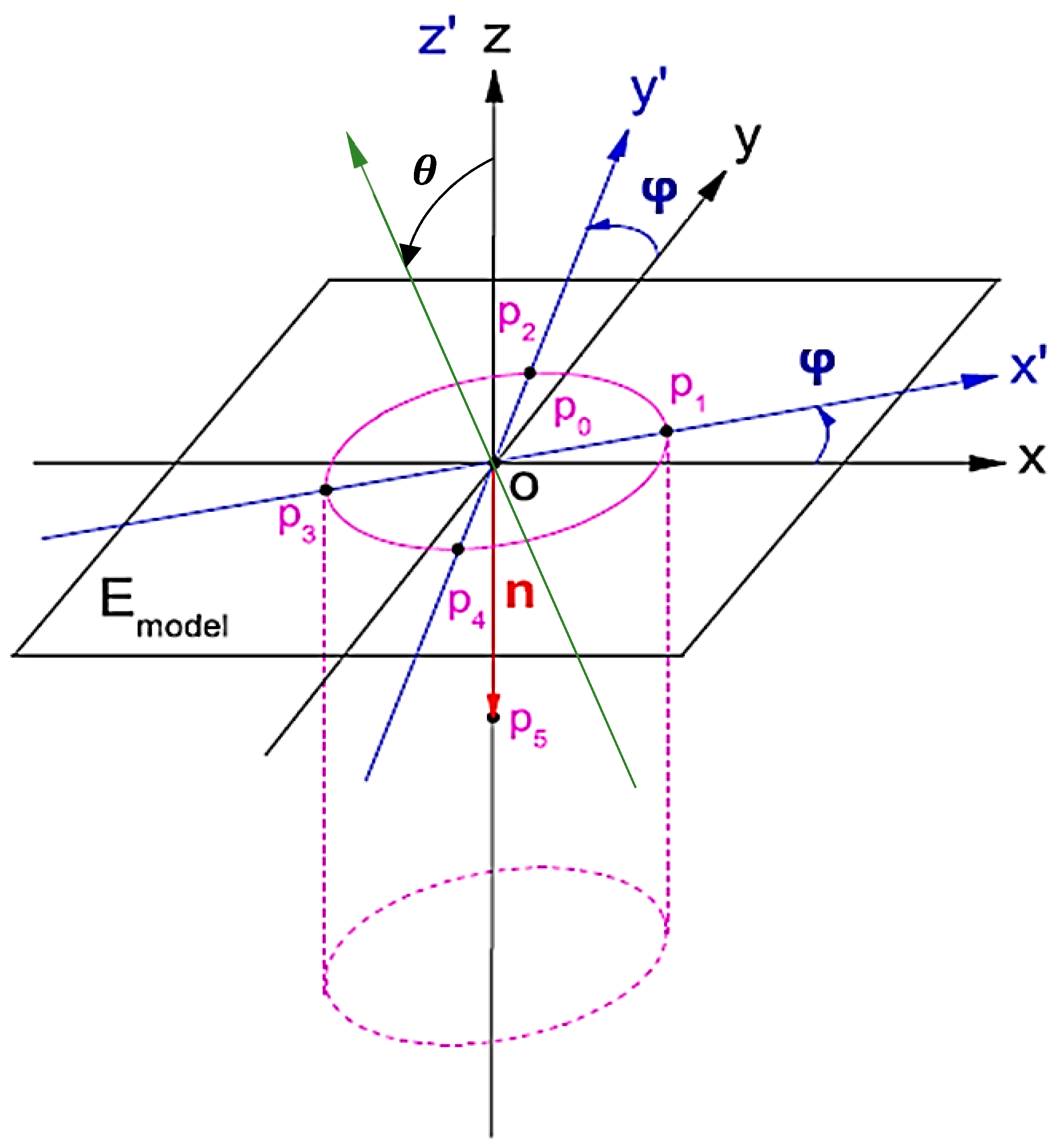Figure 2. Schematic diagram of belt-type micro hot rolling system (a) belt assembly testing schematic diagram, (b) stereo isometric view, and (c) exploded view and spare parts list.
Figure 2. Schematic diagram of belt-type micro hot rolling system (a) belt assembly testing schematic diagram, (b) stereo isometric view, and (c) exploded view and spare parts list.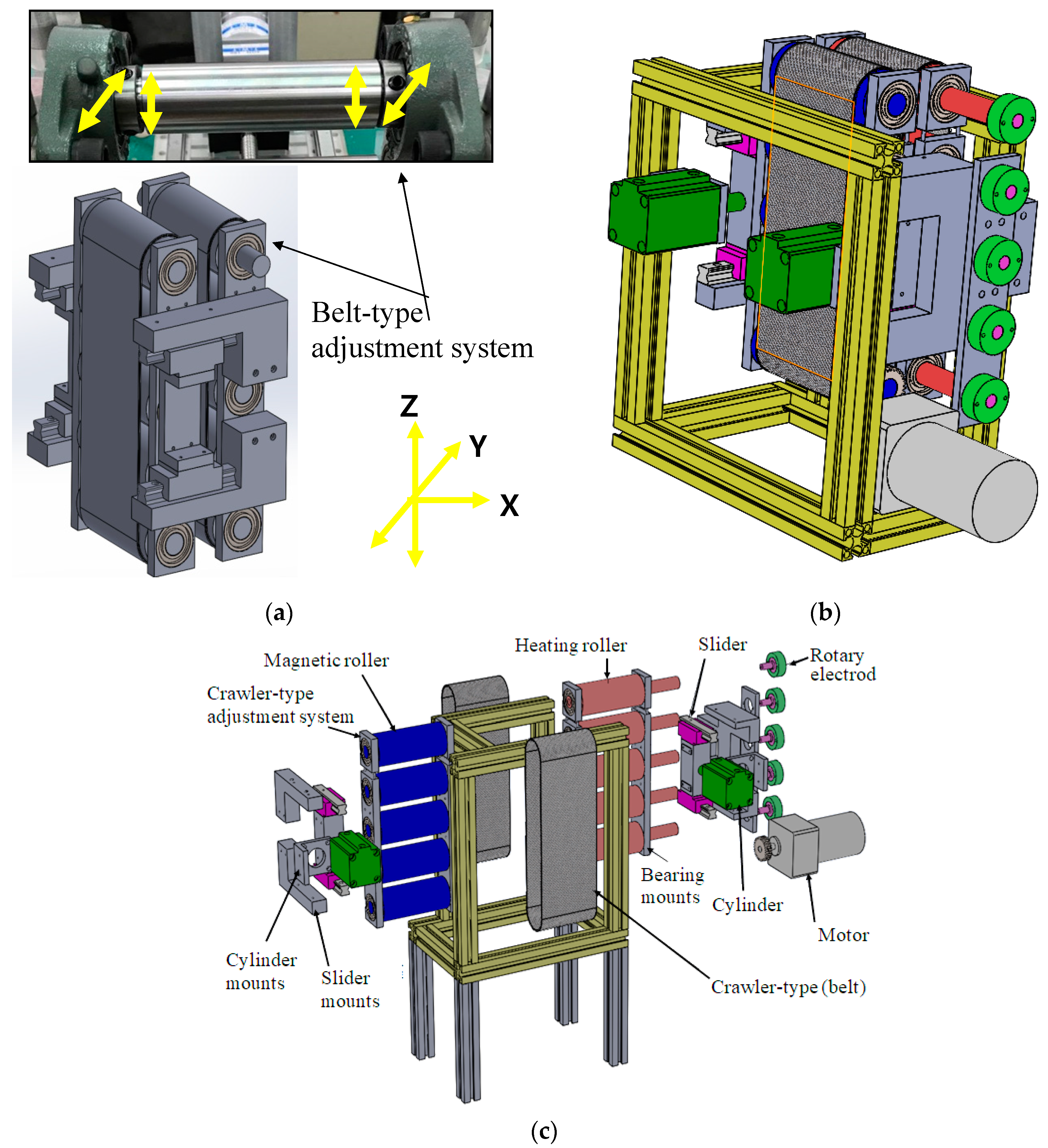Figure 3. Architecture of belt-type hot roller embossing process technology system.
Figure 3. Architecture of belt-type hot roller embossing process technology system.Figure 4. (a) Total deformation; (b) Von-Mises stress; (c) Equivalent elastic strain; (d) Directional deformation Simulation analysis diagram.
Figure 4. (a) Total deformation; (b) Von-Mises stress; (c) Equivalent elastic strain; (d) Directional deformation Simulation analysis diagram.Figure 5. Multi-layer Coated Casting: (a) exploded view, (b) combination diagram and casting operation, and (c) finished product of microstructural Belt.
Figure 5. Multi-layer Coated Casting: (a) exploded view, (b) combination diagram and casting operation, and (c) finished product of microstructural Belt.Figure 6. Fabrication of Microstructure Composite Belt by Coated Casting Technology. (a) casting PDMS; (b) insert carbon fiber fabric; (c) microstructure composite belt after integration and solidification.
Figure 6. Fabrication of Microstructure Composite Belt by Coated Casting Technology. (a) casting PDMS; (b) insert carbon fiber fabric; (c) microstructure composite belt after integration and solidification.Figure 7. Curve Graph of Tensile Test.
Figure 7. Curve Graph of Tensile Test.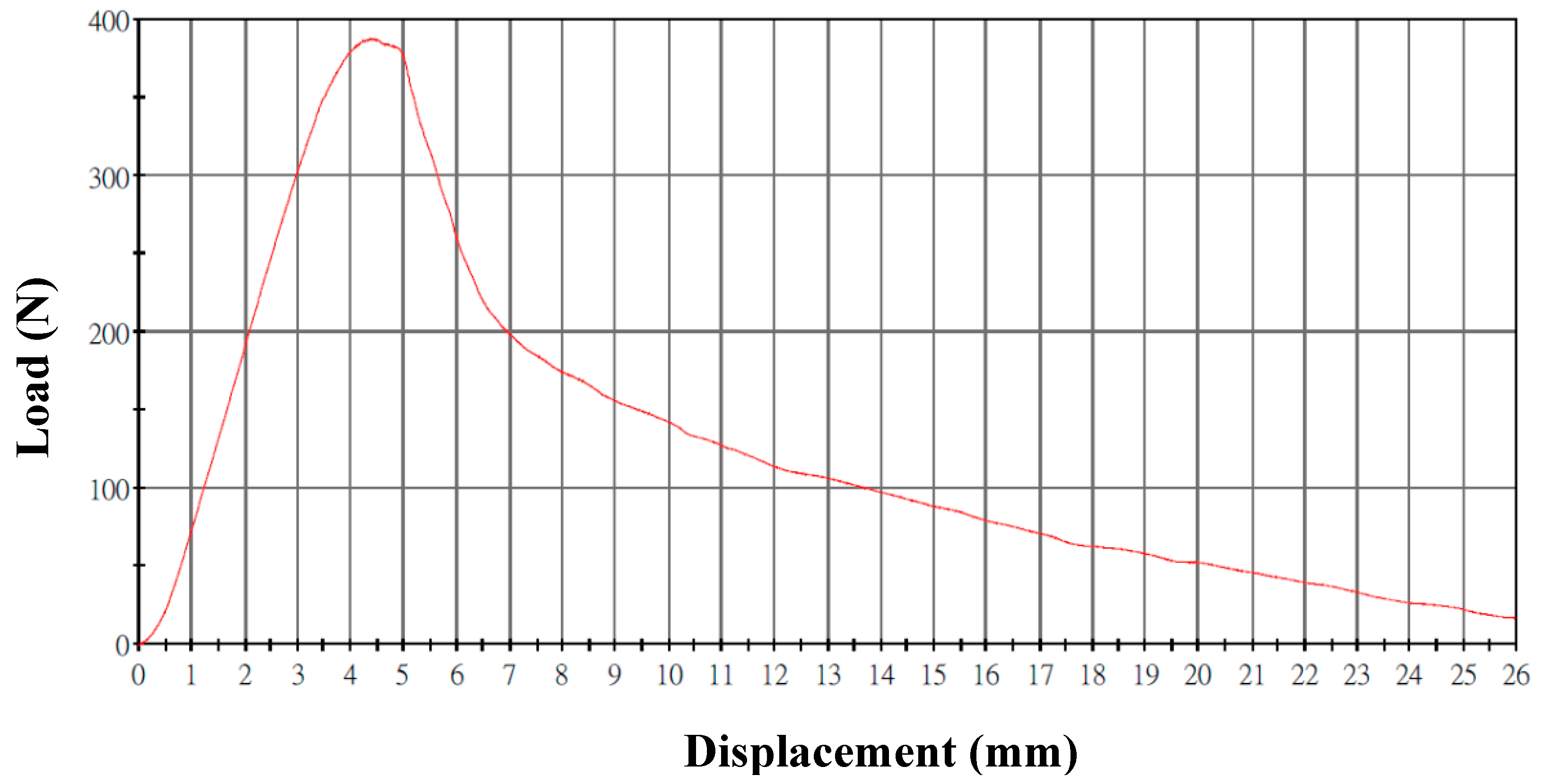Figure 8. Influence of the Weight Percentage of Alumina Powder on Maximum Tensile Load and Young’s Modulus.
Figure 8. Influence of the Weight Percentage of Alumina Powder on Maximum Tensile Load and Young’s Modulus.Figure 9. Effect of formability by unequal forming pressure and roller speed of belt-type PDMS microstructure soft mold.
Figure 9. Effect of formability by unequal forming pressure and roller speed of belt-type PDMS microstructure soft mold.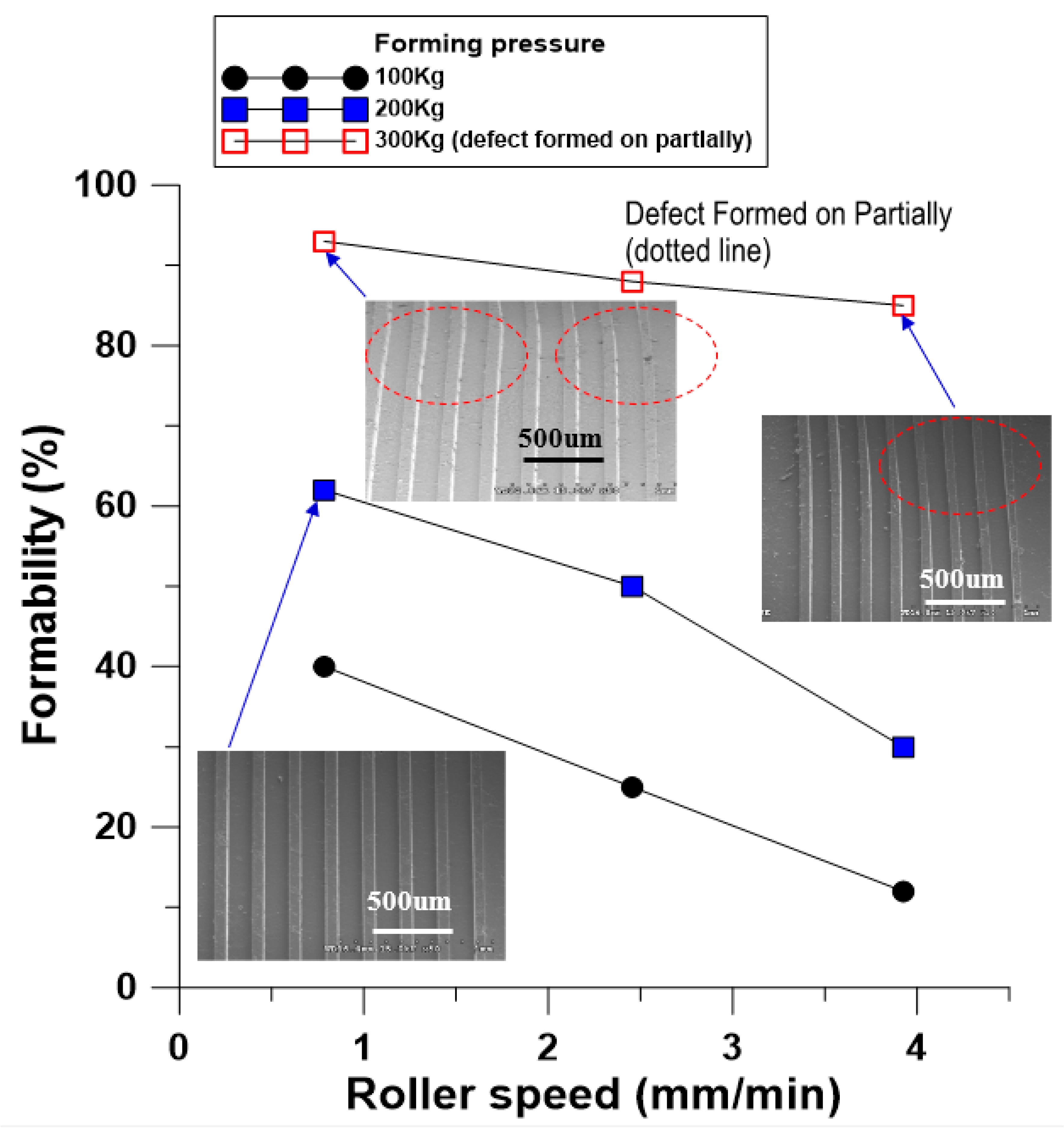Figure 10. Effect of formability by unequal forming pressure and roller speed of composite belt-type PDMS microstructure soft mold.
Figure 10. Effect of formability by unequal forming pressure and roller speed of composite belt-type PDMS microstructure soft mold.Figure 11. Scanning electron microscope (SEM) diagram of belt microstructure array.
Figure 11. Scanning electron microscope (SEM) diagram of belt microstructure array.Figure 12. Extrusion film and belt rolling complementary microstructural array. (a) Numerical simulation prediction diagram and (b) SEM diagram.
Figure 12. Extrusion film and belt rolling complementary microstructural array. (a) Numerical simulation prediction diagram and (b) SEM diagram.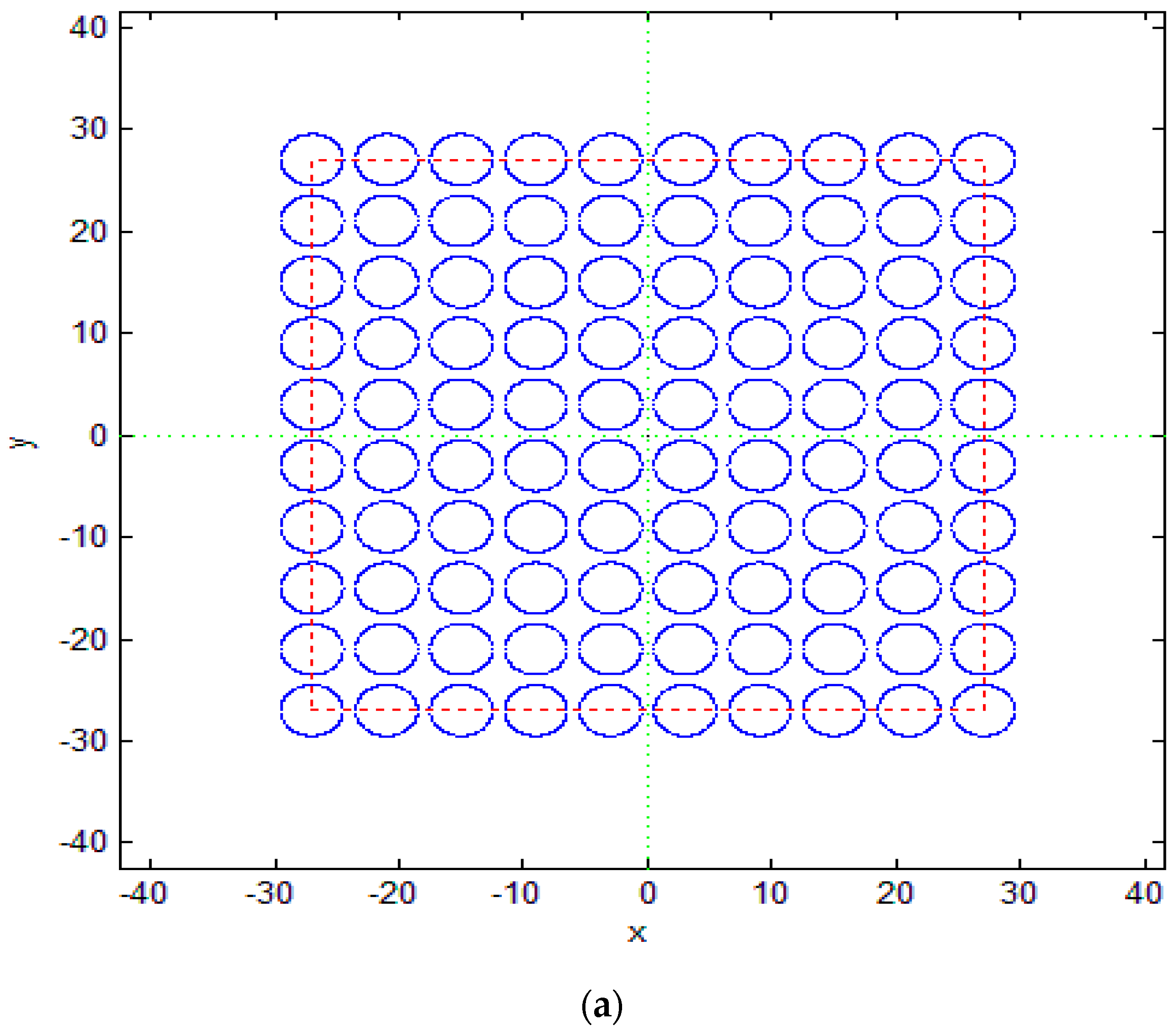Figure 13. Simulation analysis and in-depth SEM diagram under conditions of belt rolling $φ$ = 5°, $θ$ = 0.04°.
Figure 13. Simulation analysis and in-depth SEM diagram under conditions of belt rolling $φ$ = 5°, $θ$ = 0.04°.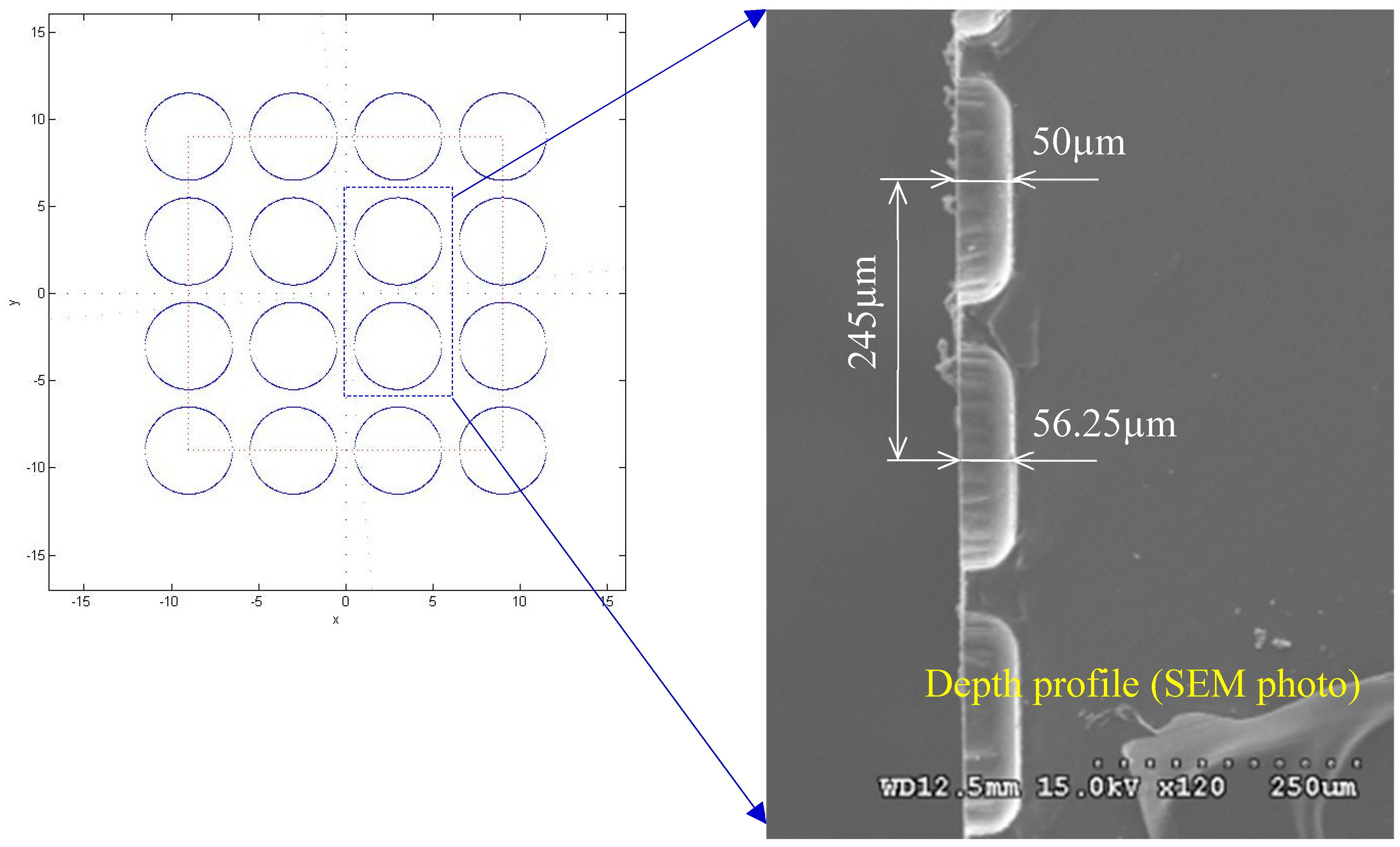Figure 14. Numerical simulation results of single microstructural forming angle $φ$ = 30°, $θ$ = 20°.
Figure 14. Numerical simulation results of single microstructural forming angle $φ$ = 30°, $θ$ = 20°.Figure 15. Numerical simulation results of array microstructural forming angle $φ$ = 45°, $θ$ = 45°.
Figure 15. Numerical simulation results of array microstructural forming angle $φ$ = 45°, $θ$ = 45°.Figure 16. Influence of unequal roller embossing pressure on the accuracy of the special forming angle.
Figure 16. Influence of unequal roller embossing pressure on the accuracy of the special forming angle.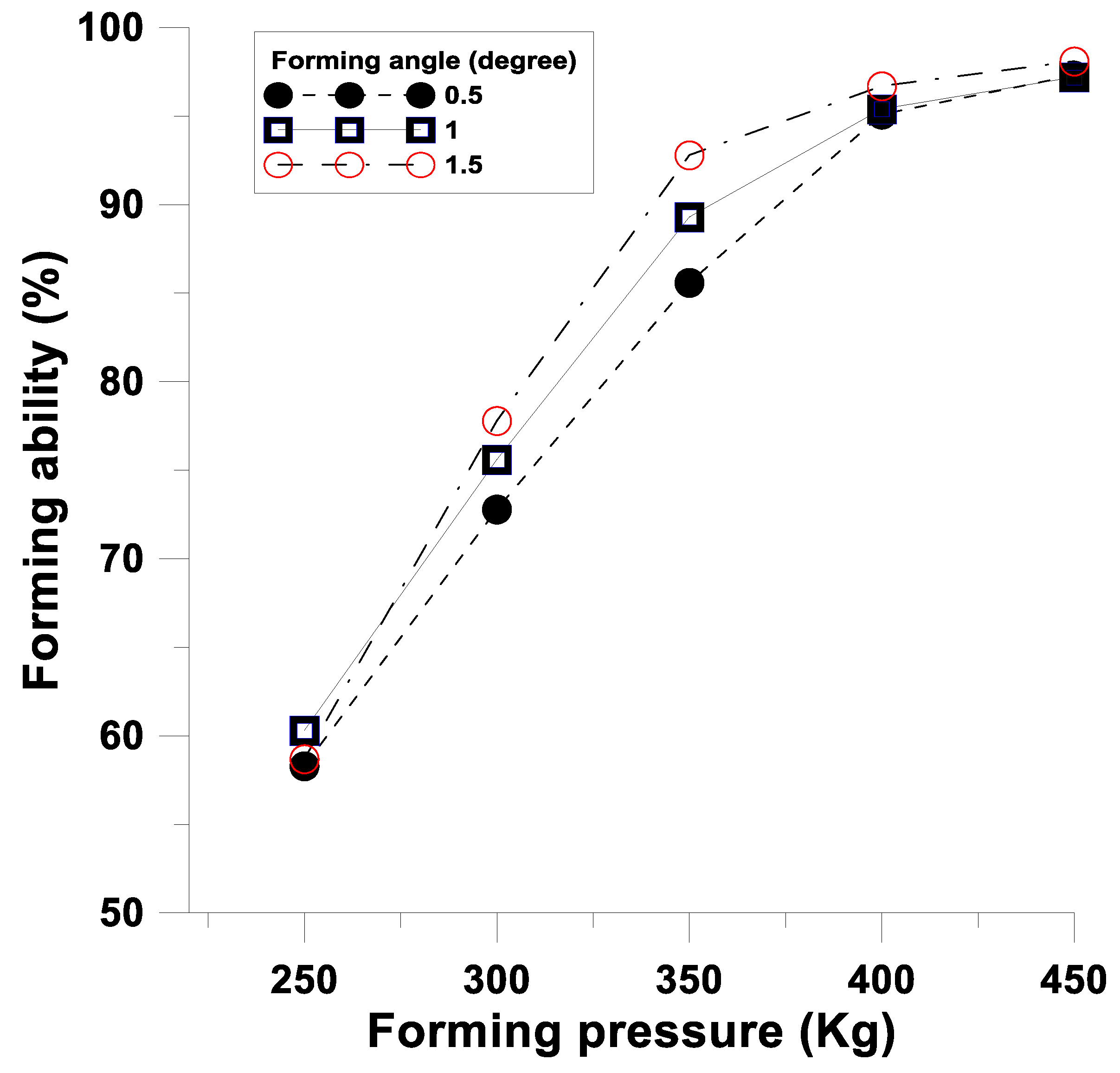Table 1. Material parameters of soft mold simulation.
Table 1. Material parameters of soft mold simulation.
MaterialsDensity (Kg·m−3)Young’s Modulus (Gpa)Poisson’s Ratio
Aluminum alloy2770710.33
PDMS (10:1)9651.720.495
Table 2. Polydimethylsiloxane (PDMS) mechanical properties test results of different circular arc radiuses.
Table 2. Polydimethylsiloxane (PDMS) mechanical properties test results of different circular arc radiuses.
(mm)
Push Distance (mm)The Mixing Ratio of Base and Curing Agent (A:B)Young’s Modulus
(MPa)
Ultimate Tensile Strength (MPa)
2855:11.225721.18493
105:11.345540.97491
510:11.414271.08201
1010:11.564881.07021
3355:11.176231.73221
105:11.739831.53826
510:11.341451.13452
1010:11.392421.08797
0010:11.963248.30731
Table 3. Mixed test results of PDMS with mixtures of 10~40% alumina powders.
Table 3. Mixed test results of PDMS with mixtures of 10~40% alumina powders.
Weight Percentage (Al2O3)Maximum Tensile Load (N)Young’s Modulus (MPa)
10%20.8530.97122
20%30.8341.98342
30%34.6032.62309
40%50.8123.42849
Table 4. 30% alumina powder PDMS composite carbon fiber belt.
Table 4. 30% alumina powder PDMS composite carbon fiber belt.
SampleMaximum Tensile Load (N)Young’s Modulus (MPa)
A386.34521048.9282
B376.34921086.3820
C380.78401076.2809
D387.33421102.3927
E384.12031089.2815
Average382.98651080.6530
Table 5. Relationship between the relative extrusion output and the screw speed of the extruder.
Table 5. Relationship between the relative extrusion output and the screw speed of the extruder.
Temperature (Degrees of Temperature, °C)Screw Speed (Revolution Per Minute, RPM)Polycarbonate Extrusion Weight (g/min)
27515010.833
25020.5
35028.667
45038.667